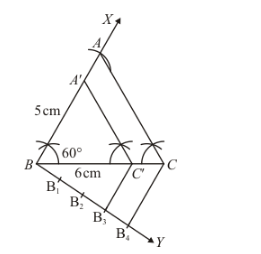# Draw a triangle ABC with side BC = 6 cm,`
Question:

Draw a triangle $A B C$ with side $B C=6 \mathrm{~cm}, A B=5 \mathrm{~cm}$ and $\angle A B C=60^{\circ}$. Then construct a triangle whose sides are $\frac{3}{4}$ time the corresponding sides of $\triangle A B C$.

Solution:

We have to draw a triangle $A B C$ with $B C=6 \mathrm{~cm}, A B=5 \mathrm{~cm}, \angle A B C=60^{\circ}$

Then we have to construct a similar triangle with side $\frac{3}{4}$ of $\triangle A B C$

Steps of construction

1. Draw a line segment2. At B drawso that a ray BX is made

3. Keep compass at B and mark an arc of 5 cm at ray BX and name that point as A4. Join $A C$ to make $\triangle A B C$

5. Draw a ray $\overrightarrow{\mathrm{BY}}$ making an acute angle with $\mathrm{BC}$.

6. Mark 4 points, $B_{1}, B_{2}, B_{3}$, and $B_{4}$ along $B Y$ such that $B B_{1}=B_{1} B_{2}=B_{2} B_{3}=B_{3} B_{4}$.

7. Join CB4

8. Through the point B3, draw a line parallel to B4C by making an angle equal to ∠BB4C, intersecting BC at C′.

9. Through the point C′, draw a line parallel to AC, intersecting BA at A′.

Thus, ΔA′BC′ is the required triangle.